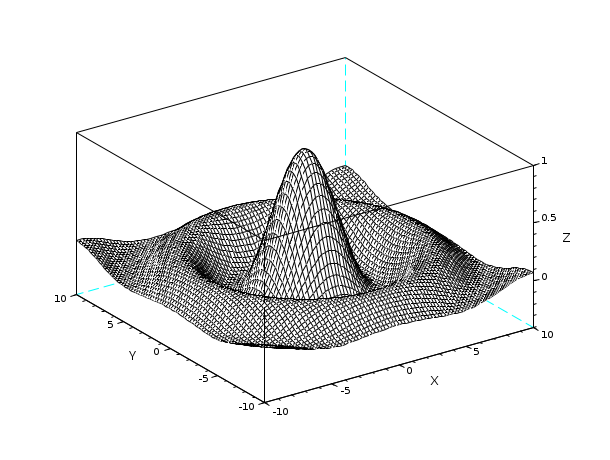Scilab Home page | Wiki | Bug tracker | Forge | Mailing list archives | ATOMS | File exchange
Scilab-Branch-6.1-GIT
Change language to: Français - Português - 日本語 - Русский
Scilab Help >> Special Functions > sinc

# sinc

sinc function

### Syntax

`t=sinc(x)`

### Arguments

x

a real or complex vector or matrix, in radians.

t

a real or complex vector or matrix.

### Description

If `x` is a vector or a matrix, `t=sinc(x)` is the vector or matrix such that `t(i)=sin(x(i))/x(i)` if `x(i)~=0` and `t(i)=1` if `x(i)==0.`

### Examples

```x=linspace(-10,10,3000);
plot2d(x,sinc(x))``````[X,Y] = meshgrid(-10:0.25:10,-10:0.25:10);
f = sinc(sqrt((X).^2+(Y).^2));
mesh(X,Y,f);```